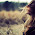### Single Number

Given an array of integers, every element appears twice except for one. Find that single one.
Note:
Your algorithm should have a linear runtime complexity. Could you implement it without using extra memory?

### Analysis:

The requirement is O(n) time and O(1) space.
Thus, the  "first sort and then find " way is not working.
Also the "hash map" way is not working.

Since we can not sort the array, we shall find a cumulative way, which is not about the ordering.

XOR is a good way, we can use the property that A XOR A = 0, and A XOR B XOR A = B.

So, the code becomes extremely easy.

### Code(C++):

class Solution {
public:
int singleNumber(int A[], int n) {
// IMPORTANT: Please reset any member data you declared, as
// the same Solution instance will be reused for each test case.
int res = A;
for (int i=1;i<n;i++){
res = res ^ A[i];
}
return res;
}
};


### Code (Python):

class Solution:
# @param A, a list of integer
# @return an integer
def singleNumber(self, A):
res = 0
for a in A:
res = res ^ a
return res


#### 1 comment:

1.This comment has been removed by the author.